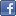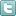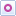EE Times-India > EDA/IP

EDA/IP# Giving Laplace transform a second look

Posted: 31 Mar 2015Print Version

Keywords:CERN  Laplace transform  Fourier transform

The Laplace transform is an integral transform that is arguably second only to the Fourier transform in terms of application in solving physical problems. The Laplace transform is particularly useful in solving linear ordinary differential equations such as those arising in the analysis of electronic circuits. In all its complexity, the Laplace transform offers an alternative functional description that often simplifies the process of analysing the behaviour of the system.

Can you find the error(s), if any, in the following mathematical derivation that applies the Laplace transform? Begin by expressing the function to be transformed, g(t), in the form of the square of f(t), or g(t) = f 2(t). Then by the definition of the Laplace transform,Apply the integration by parts formula for definite integrals:where u and v are both functions of t, and ti and tf are the integration limits of t. ChooseandCombining these in the formula,where the integration limits that applied to t are converted to f. To convert them, apply the condition on f (t) thatThus,The integration is with respect to f, not t, and consequently the limits of integration have been changed to f (0) and f (∞), as indicated. When the integral is taken and the resulting expression is simplified,Therefore, the Laplace transform of all functions of the form g(t) are G(s) = 0.

Nobody who has worked with Laplace transforms would accept this result as valid. Where is the error?

Hint: one error is in the line that readsTo find v = v(t), the indefinite integral of the integrand f(t)?e?st must be taken, then the limits of integration applied to it. The error is in equating expressions that are not necessarily equal:If v were equal to F(s), then v(t) is constant with respect to F(s) and dv/dt = 0, which is consistent with the faulty conclusion.

Erik Margan, sensor-electronics designer of the ATLAS experiment of the Large Hadron Collider (LHC) at CERN found additional errors. Can you identify them?

- Dennis Feucht
EDN

 Related Articles Editor's Choice
Comment on "Giving Laplace transform a second lo..."

Top Ranked Articles

WebinarsVisit Asia Webinars to learn about the latest in technology and get practical design tips.

Search EE Times India
Services

Go to topConnect on FacebookFollow us on TwitterFollow us on Orkut
﻿